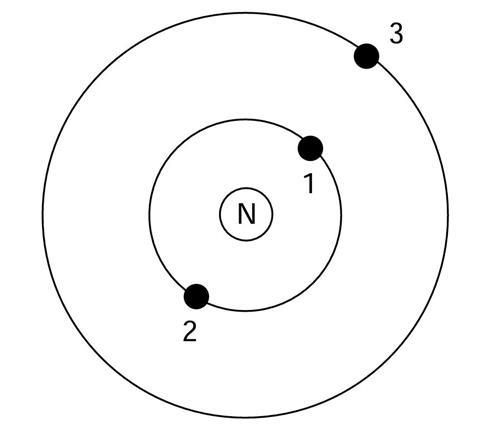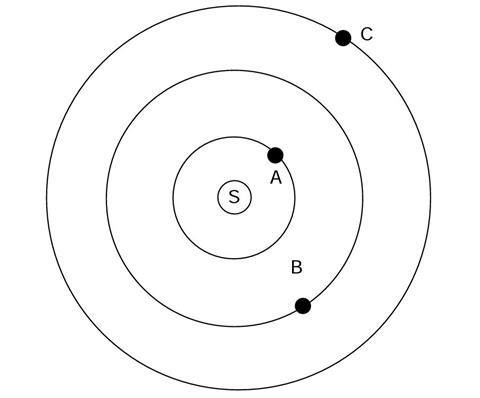One analogy that is commonly used is that ‘the atom is like a tiny solar system’

However, without help, many students have difficulties recognising which aspects of an analogy they are meant to attend to. Students may also be less familiar with the analogue than is assumed.

## The atom and the solar system

The diagram shows a simple model of an atom.N is the nucleus, and there are three electrons, labelled 1, 2 and 3.

The electrons are attracted to the nucleus.

The diagram shows a simple model of a solar system.

S is the sun, and there are three planets, labelled A, B and C.Below are some questions about the model of the atom, and the solar system, shown in the diagrams.

1. What type of force attracts the electrons towards the nucleus?
2. Is electron 3 attracted to the nucleus by a stronger force, a weaker force, or the same size force as electron 1?
1. Why do you think this?
3. Which statement do you think is correct ?
1. The force attracting the nucleus to electron 2 is larger than the force attracting electron 2 to the nucleus.
2. The force attracting the nucleus to electron 2 is the same size as the force attracting electron 2 to the nucleus.
3. The force attracting the nucleus to electron 2 is smaller than the force attracting electron 2 to the nucleus.
4. There is no force acting on the nucleus attracting it to electron 2.
5. Why do you think this?
4. Is there any force between electron 1 and electron 3?
1. Why do you think this?
5. What type of force attracts the planets towards the sun?
6. Is planet C attracted to the sun by a stronger force, a weaker force, or the same size force as planet A?
1. Why do you think this is?
7. Which statement do you think is correct
1. The force attracting the sun to planet B is larger than the force attracting planet B to the sun.
2. The force attracting the sun to planet B is the same size as the force attracting planet B to the sun.
3. The force attracting the sun to planet B is smaller than the force attracting planet B to the sun.
4. There is no force acting on the sun attracting it to planet B.
5. Why do you think this is?
8. Is there any force between planet A and planet C?
1. Why do you think this is?

## Comparing the atom with the solar system

Look at the models shown in the diagrams, and try to think of ways in which the atom and the solar system are similar, and ways in which they are different.

List the similarities and differences you can think of.

### The atom and the solar system

1. Electrical
2. The force attracting electron 3 is weaker – as it is a greater distance from the nucleus.
3. The force attracting the nucleus to the electron is the same size as the force attracting the electron to the nucleus – the forces between two bodies always act with the same magnitude (size) on both (‘action’ = ‘reaction’).
4. Yes (electrical) – they repel as they both have negative charges.
5. Gravitational
6. The force attracting planet C is weaker – as it is a greater distance from the sun.
7. The force attracting the sun to the planet is the same size as the force attracting the planet to the sun – the forces between two bodies always act with the same magnitude (size) on both (‘action’ = ‘reaction’).
8. Yes (gravitational) – they attract as they both have mass.

### Comparing the atom with the solar system

Note: that as this is an open-ended activity, other valid ideas should be welcomed. Post-16 students will normally be expected to offer more sophisticated suggestions than younger students (indicated by *).

### Similarities

• Central body
• ‘Orbiting’ * bodies (3 in the examples given)
• Most of mass of system at centre
• Orbiting bodies attract central body
• Orbiting bodies attracted by central body
• Forces act between orbiting bodies
• Nuclei and sun may have a ‘shell’ type structure

### Differences

• Size.
• Nature of attraction (electrical/gravitational); planets do not share ‘orbits’ *
• Electrons repel each other and planets attract each other; many atoms effectively identical – each solar system unique
• Solar systems evolve whereas atomic transitions are abrupt
• Electrons may effectively shield part of nuclear charge
• All electrons are identical whereas planets are each different (in mass, size, composition)
• Atoms seldom found in a free state, stable solar systems tend to be discrete
• Nucleus has ‘grain’ structure (discrete nucleons); solar system mostly in one plane (atoms approximate spherical symmetry)
• Planets may have their own satellites.
• *In post-16 courses the idea of electrons ‘orbiting’ may well have been replaced by more sophisticated ideas about orbitals, electron ‘waves’ and electron density.